## The first Nyquist Criterion¶

This article describes the 1st Nyquist Criterion, which is of central importance in the design of filters that are used for digital data transmissions. We provide expressions for the time-domain and frequency-domain and illustrate the results with Python source code.

Let us first define a python function ft which calculates the Fourier Transform of samples of a time-continuous function (refer to Approximation of the Fourier Transform). Also, define a function get_filter that can return different kinds of pulse shaping filters, which we use throughout this notebook.

def ft(samples, Fs, t0):
f = np.linspace(-Fs/2, Fs/2, len(samples), endpoint=False)
return np.fft.fftshift(np.fft.fft(samples)/Fs * np.exp(-2j*np.pi*f*t0))

def get_filter(name, T, rolloff):
def rc(t, beta):
return np.sinc(t)*np.cos(np.pi*beta*t)/(1-(2*beta*t)**2)
def rrc(t, beta):
return (np.sin(np.pi*t*(1-beta))+4*beta*t*np.cos(np.pi*t*(1+beta)))/(np.pi*t*(1-(4*beta*t)**2))

if name == 'rect':
return lambda t: (abs(t/T)<0.5).astype(int)
if name == 'triang':
return lambda t: (1-abs(t/T)) * (abs(t/T)<1).astype(float)
elif name == 'rc':
return lambda t: rc(t/T, rolloff)
elif name == 'rrc':
return lambda t: rrc(t/T, rolloff)


Let us first plot the time-domain response of these filters.

def show_filter(g, title):
t = np.linspace(-3,3, 300)
plt.plot(t, g(t))
plt.title(title)

plt.subplot(221); show_filter(get_filter('rect', 1, 0), 'Rect')
plt.subplot(222); show_filter(get_filter('triang', 1, 0), 'Triang')
plt.subplot(223); show_filter(get_filter('rc', 1, 0.5), r'RC $\alpha=0.5$')
plt.subplot(224); show_filter(get_filter('rrc', 1, 0.5), r'RRC $\alpha=0.5$')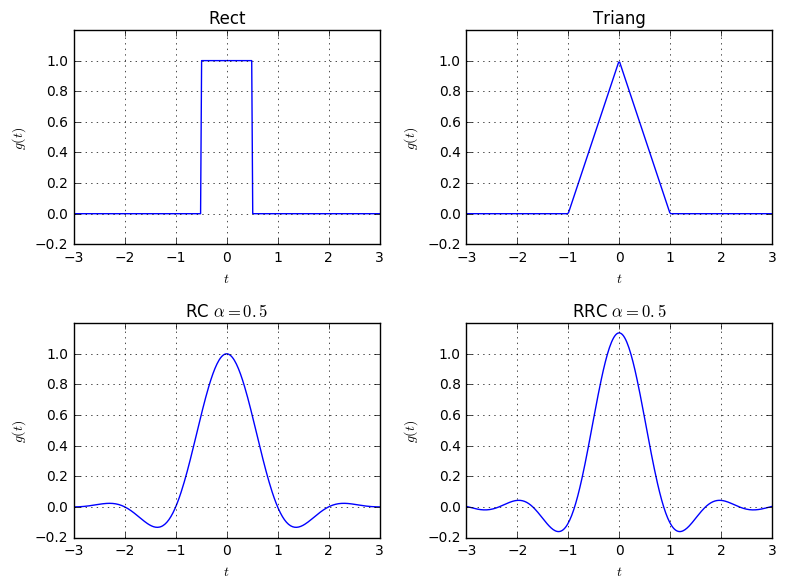The first Nyquist Criterion describes the fact, when a filter $g(t)$ does not produce Intersymbol-Interference (ISI) between adjacently transmitted symbols. In order to understand this, let us first create some pulse-shaping filter $g(t)$:

T = 1    # The distance in time between adjacent symbols
Fs = 1000  # The sampling frequency to analyze the system in the digital domain
g = get_filter('rc', T, rolloff=0.3) # Change this to e.g. 'rrc' type, to see ISI
t = np.arange(-5*T, 5*T, 1/Fs)
plt.plot(t, g(t), label='$g(t)$')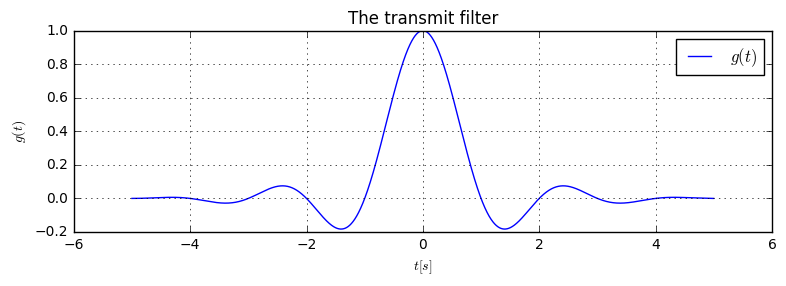Consider a digital transmission system that transmits the data $d[k]$ with an impulse $g(t)$ and symbol distance $T$. Then, the transmit signal $x(t)$ is given by

$$x(t) = \sum_{k\in\mathbb{Z}}d[k]g(t-kT)$$

We can now generate a random signal, according to this model:

# the transmit bits
b = np.array([1, 1, 0, 1, 0, 0, 1, 1, 0, 0, 0])
d = 2*b-1  # do bipolar ASK modulation (i.e. BPSK)

print ("Transmitted data: d=%s" % d)
t = np.arange(-1*T, len(d)*T+1, 1/Fs)
xt = sum(d[k]*g(t-k*T) for k in range(len(d)))  # the transmit signal
plt.plot(t, xt, '-k', lw=2, label='$x(t)$')
plt.stem(T*np.arange(len(d)), d)
for k in range(len(d)):
plt.plot(t, d[k]*g(t-k*T), "b-", **kwargs)

Transmitted data: d=[ 1  1 -1  1 -1 -1  1  1 -1 -1 -1]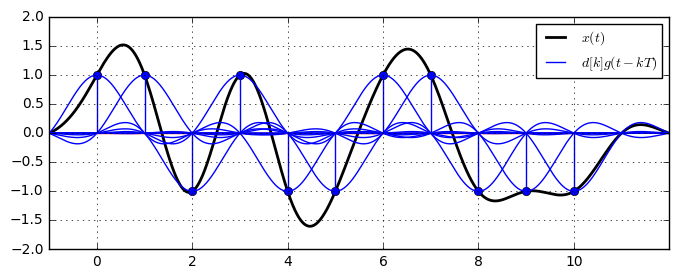Now, the first Nyquist theorem requires that the signal value $x(kT)$ at the sample time $kT$ of the transmit symbols does only depend on the transmit symbol for the time $kT$. Mathematically, we can describe this as follows: $$x(kT)=d[k].$$ See, how this property is fulfilled in the above plot: The black line leads exactly through the transmitted data points (blue dots) (You can change the filter to e.g. RRC and see the signal is not ISI-free anymore). We can directly derive the following property of the transmit filter $g(t)$

$$g(kT) = \begin{cases}1 & k=0\\0 & k\neq 0\end{cases},$$

meaning the filter must have zeros at the symbol times, except in its center. If we insert this property into the expression for $x(t)$, we directly get $$x(kT)=\sum_{k'\in\mathbb{Z}}d[k']g((k'-k)T)=d[k],$$

proving the ISI-freeness of the signal. We can equivalently express the requirement on the filter as $$g(t)\sum_{k\in\mathbb{Z}}\delta(t-kT)=\delta(t),$$ which is illustrated below.

t = np.arange(-10*T, 10*T, 1/Fs)
pulse_train = np.zeros(len(t))
pulse_train[::int(T*Fs)] = 1   # Create the impulse train
plt.plot(t, g(t), label='$g(t)$')
plt.plot(t, pulse_train, label='$\sum_{k\in\mathbb{Z}}\delta(t-kT)$')
plt.plot(t, g(t)*pulse_train, label='$g(t)\sum_{k\in\mathbb{Z}}\delta(t-kT)$')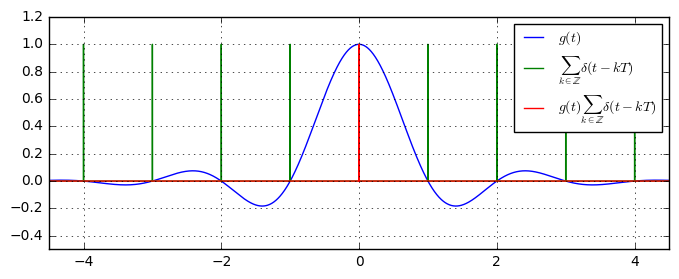As we can see, the 1st Nyquist criterion is fulfilled, since the red line only is non-zero at $t=0$. If we transform requirement from the time-domain into the frequency domain, we get

$$G(f)*\frac{1}{T}\sum_{k\in\mathbb{Z}}\delta(f-k/T) = 1.$$

Expressing the convolution with the Diracs as frequency-shifts, we end up with the common expression for the 1st Nyquist criterion:

$$\sum_{k\in\mathbb{Z}}G(f-k/T)=T,$$

i.e. a summation of the filter, periodically shifted by $1/T$ must result in a constant value. Let us write this expression into the source code:

g_times_pulse = g(t) * pulse_train
G = ft(g(t), Fs, min(t))
G_times_pulse = ft(g_times_pulse, Fs, min(t)) * Fs *T # Normalization constant
f = np.linspace(-Fs/2, Fs/2, len(G_times_pulse))

# frequency bin distance of the Fourier Transform approximation
df_per_sample = Fs/len(G_times_pulse)

plt.subplot(121)
plt.plot(t, g_times_pulse, 'k-', lw=2, label=r'$g(t)\sum_{k\in\mathbb{Z}}\delta(t-kT)$')
plt.plot(t, g(t), 'b-', label='$g(t)$')
plt.subplot(122)
plt.plot(f, abs(G_times_pulse), 'k-', lw=2, label=r'$\mathcal{F}\{\delta(t)\}$')
for k in range(-9, 9):
plt.plot(f, np.real(np.roll(G, int(k*1/(df_per_sample*T)))), 'b', label=None if k else r'$G(f-\frac{k}{T})$')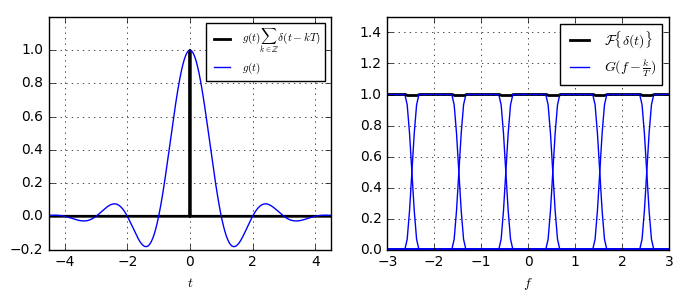As we can see, for the chosen pulse, the 1st Nyquist Criterion is fulfilled. Its periodically shifted frequency responses sum up to a constant and in the time domain, sampling at the symbol times yields a single peak at the symbol position.

In the code below, we check the 1st Nyquist Criterion for different choices of filters.

def NyquistFirst(g):
plt.subplot(131)
t = np.arange(-20*T, 20*T, 1/Fs)
pulse_train = np.zeros(len(t))
pulse_train[::int(Fs*T)] = 1
g_times_pulse = g(t)*pulse_train

plt.plot(t, g(t))
plt.plot(t, g_times_pulse)

plt.subplot(132)
f = np.linspace(-Fs/2, Fs/2, len(t))
G = ft(g(t), Fs, min(t)) # calculate spectrum of g(t)
G_times_pulse = ft(g_times_pulse, Fs, min(t)) * Fs * T
df_per_sample = Fs/len(t)
for k in range(-50,50):
Gshift = np.roll(G, int(k*1/(df_per_sample*T)))  # calculate a frequency-shift by k/T
plt.plot(f, np.real(Gshift), '-')
plt.plot(f, abs(G_times_pulse), 'k', lw=2)

plt.subplot(133)
plt.plot(f, abs(G))
BN = 1/(2*T)
plt.axvline(BN)
plt.axvline(-BN)

NyquistFirst(get_filter('rrc', T, 0.5)) # change to rc filter to see ISI-free

NyquistFirst(get_filter('rect', T, 1))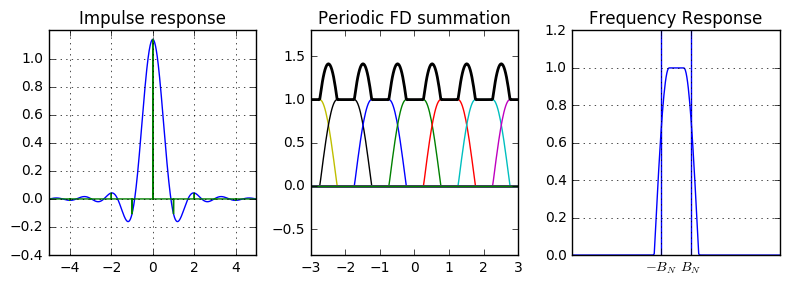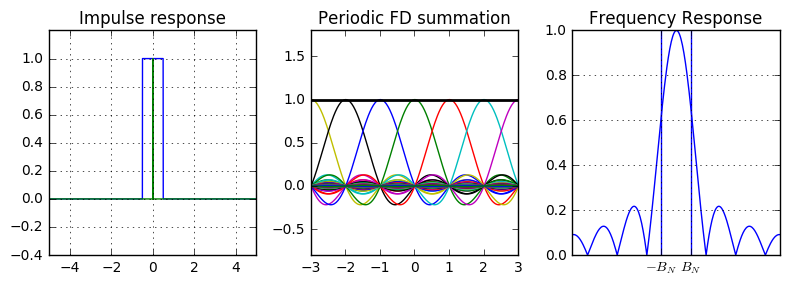For example, the (Root raised cosine) RRC filter does not fulfill the 1st Nyquist Criterion. This is both seen in the time-domain plot whereas also in the periodic summation in the frequency domain. Note that its amplitude response follows that of a square root of the raised cosine filter.

The rectangular filter fulfills the 1st Nyquist Criterion. But, due to its abrupt changes in the time domain, its bandwidth is much larger than that of the e.g. raised cosine.

The minimum achievable bandwidth for ISI-free transmission is the Nyquist-Bandwidth $B_N=1/(2T)$. This bandwidth is achieved by the ideal lowpass. However, this particular filter can hardly be realized due to its very wide response in the time domain.

### Conclusion¶

In this article, we have derived the 1st Nyquist Criterion, which ensures an ISI-free signal. In the time domain, the 1st Nyquist Criterion can be expressed as

$$g(kT) = \begin{cases}1 & k=0 \\ 0 &k; \neq 0\end{cases}.$$

In the frequency domain, this property corresponds to the requirement of

$$\sum_{k\in\mathbb{Z}}G(f-\frac{k}{T})=T,$$

with $G(f)=\mathcal{F}\{g(t)\}$.